International
Tables for
Crystallography
Volume A
Space-group symmetry
Edited by M. I. Aroyo

International Tables for Crystallography (2015). Vol. A, ch. 1.4, pp. 56-59

Section 1.4.2.5. Space-group diagrams

M. I. Aroyo,a G. Chapuis,b B. Souvignierd and A. M. Glazerc

1.4.2.5. Space-group diagrams

| top | pdf |

In the space-group tables of Chapter 2.3, for each space group there are at least two diagrams displaying the symmetry (there are more diagrams for space groups of low symmetry). The symmetry-element diagram displays the location and orientation of the symmetry elements of the space group. The general-position diagrams show the arrangement of a set of symmetry-equivalent points of the general position. Because of the period­icity of the arrangements, the presentation of the contents of one unit cell is sufficient. Both types of diagrams are orthogonal projections of the space-group unit cell onto the plane of projection along a basis vector of the conventional crystallographic coordinate system. The symmetry elements of triclinic, monoclinic and orthorhombic groups are shown in three different projections along the basis vectors. The thin lines outlining the projection are the traces of the side planes of the unit cell.

Detailed explanations of the diagrams of space groups are found in Section 2.1.3.6. In this section, after a very brief introduction to the diagrams, we will focus mainly on certain important but very often overlooked features of the diagrams.

Symmetry-element diagram

The graphical symbols of the symmetry elements used in the diagrams are explained in Section 2.1.2. The heights along the projection direction above the plane of the diagram are indicated for rotation or screw axes and mirror or glide planes parallel to the projection plane, for rotoinversion axes and inversion centres. The heights (if different from zero) are given as fractions of the shortest translation vector along the projection direction. In Fig. 1.4.2.6(left) the symmetry elements of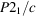(unique axis b, cell choice 1) are represented graphically in a projection of the unit cell along the monoclinic axis b. The directions of the basis vectors c and a can be read directly from the figure. The origin (upper left corner of the unit cell) lies on a centre of inversion indicated by a small open circle. The black lenticular symbols with tails represent the twofold screw axes parallel to b. The c-glide plane at heightalong b is shown as a bent arrow with the arrowhead pointing along c.Figure 1.4.2.6 | top | pdf |Symmetry-element diagram (left) and general-position diagram (right) for the space group, No. 14 (unique axis b, cell choice 1).

The crystallographic symmetry operations are visualized geometrically by the related symmetry elements. Whereas the symmetry element of a symmetry operation is uniquely defined, more than one symmetry operation may belong to the same symmetry element (cf. Section 1.2.3). The following examples illustrate some important features of the diagrams related to the fact that the symmetry-element symbols that are displayed visualize all symmetry operations that belong to the element sets of the symmetry elements.

Examples

• (1) Visualization of the twofold screw rotations of(Fig. 1.4.2.6). The second coset of the decomposition ofwith respect to its translation subgroup shown in Table 1.4.2.6is formed by the infinite set of twofold screw rotations represented by the coordinate triplets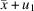,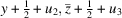(where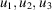are integers). To analyse how these symmetry operations are visualized, it is convenient to consider two special cases:

 (i)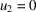, i.e.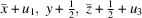=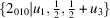; these operations correspond to twofold screw rotations around the infinitely many screw axes parallel to the line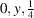, i.e. around the lines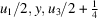. The symbols of the symmetry elements (i.e. of the twofold screw axes) located in the unit cell at;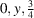;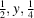;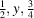(and the translationally equivalent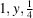and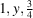) are shown in the symmetry-element diagram (Fig. 1.4.2.6); (ii)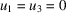, i.e.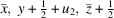=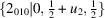; these symmetry operations correspond to screw rotations around the linewith screw components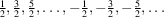, i.e. with a screw componentto which all lattice translations parallel to the screw axis are added. These operations, infinite in number, share the same geometric element, i.e. they form the element set of the same symmetry element, and geometrically they are represented just by one graphical symbol on the symmetry-element diagrams located exactly at. (iii) The rest of the symmetry operations in the coset, i.e. those with the translation parts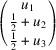, are combinations of the two special cases above.

• (2) Inversion centres of(Fig. 1.4.2.6). The element set of an inversion centre consists of only one symmetry operation, viz. the inversion through the point located at the centre. In other words, to each inversion centre displayed on a symmetry-element diagram there corresponds one symmetry operation of inversion. The infinitely many inversions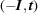=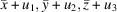=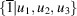ofare located at points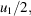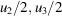. Apart from translational equivalence, there are eight centres located in the unit cell: four at y = 0, namely at 0, 0, 0;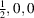;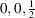;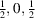and four at heightof b. It is important to note that only inversion centres at y = 0 are indicated on the diagram.

A similar rule is applied to all pairs of symmetry elements of the same type (such as e.g. twofold rotation axes, planes etc.) whose heights differ byof the shortest lattice direction along the projection direction. For example, the c-glide plane symbol in Fig. 1.4.2.6with the fractionnext to it represents not only the c-glide plane located at heightbut also the one at height.

• (3) Glide reflections visualized by mirror planes. As discussed in Section 1.2.3, the element set of a mirror or glide plane consists of a defining operation and all its coplanar equivalents (cf. Table 1.2.3.1). The corresponding sym­metry element is a mirror plane if among the infinite set of the coplanar glide reflections there is one with zero glide vector. Thus, the symmetry element is a mirror plane and the graphical symbol for a mirror plane is used for its representation on the symmetry-element diagrams of the space groups. For example, the mirror plane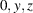shown on the symmetry-element diagram of Fmm2 (42), cf. Fig. 1.4.2.3, represents all glide reflections of the element set of the defining operation[symmetry operation (4) of the general-position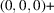set, cf. Fig. 1.4.2.2], including the n-glide reflection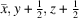[entry (4) of the general-position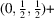set]. In a similar way, the graphical symbols of the mirror planes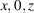also represent the n-glide reflections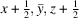[entry (3) of the general-position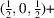set] of Fmm2.

General-position diagram

The graphical presentations of the space-group symmetries provided by the general-position diagrams consist of a set of general-position points which are symmetry equivalent under the symmetry operations of the space group. Starting with a point in the upper left corner of the unit cell, indicated by an open circle with a sign +', all the displayed points inside and near the unit cell are images of the starting point under some symmetry operation of the space group. Because of the one-to-one correspondence between the image points and the symmetry operations, the number of general-position points in the unit cell (excluding the points that are equivalent by integer translations) equals the multiplicity of the general position. The coordinates of the points in the projection plane can be read directly from the diagram. For all systems except cubic, only one parameter is necessary to describe the height along the projection direction. For example, if the height of the starting point above the projection plane is indicated by a +' sign, then signs +', −' or their combinations with fractions (e.g.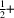,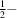etc.) are used to specify the heights of the image points. A circle divided by a vertical line represents two points with different coordinates along the projection direction but identical coordinates in the projection plane. A comma `,' in the circle indicates an image point obtained by a symmetry operation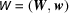of the second kind [i.e. with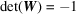, cf. Section 1.2.2].

Example

The general-position diagram of(unique axis b, cell choice 1) is shown in Fig. 1.4.2.6(right). The open circles indicate the location of the four symmetry-equivalent points of the space group within the unit cell along with additional eight translation-equivalent points to complete the presentation. The circles with a comma inside indicate the image points generated by operations of the second kind – inversions and glide planes in the present case. The fractions and signs close to the circles indicate their heights in units of b of the symmetry-equivalent points along the monoclinic axis. For example,is a shorthand notation for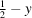.

Notes:

• (1) The close relation between the symmetry-element and the general-position diagrams is obvious. For example, the points shown on the general-position diagram are images of a general-position point under the action of the space-group symmetry operations displayed by the corresponding symmetry elements on the symmetry-element diagram. With some practice each of the diagrams can be generated from the other. In a number of texts, the two diagrams are considered as completely equivalent descriptions of the same space group. This statement is true for most of the space groups. However, there are a number of space groups for which the point configuration displayed on the general-position diagram has higher symmetry than the generating space group (Suescun & Nespolo, 2012; Müller, 2012). For example, consider the diagrams of the space group P2, No. 3 (unique axis b, cell choice 1) shown in Fig. 1.4.2.7. It is easy to recognise that, apart from the twofold rotations, the point configuration shown in the general-position diagram is symmetric with respect to a reflection through a plane containing the general-position points, and as a result the space group of the general-position configuration is of P2/m type, and not of P2. There are a number of space groups for which the general-position diagram displays higher space-group symmetry, for example: P1,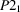, P4mm, P6 etc. The analysis of the eigensymmetry groups of the general-position orbits results in a systematic procedure for the determination of such space groups: the general-position diagrams do not reflect the space-group symmetry correctly if the general-position orbits are non-characteristic, i.e. their eigensymmetry groups are supergroups of the space groups. (An introduction to terms like eigensymmetry groups, characteristic and non-characteristic orbits, and further discussion of space groups with non-characteristic general-position orbits are given in Section 1.4.4.4.)Figure 1.4.2.7 | top | pdf |Symmetry-element diagram (left) and general-position diagram (right) for the space group P2, No. 3 (unique axis b, cell choice 1).
• (2) The graphical presentation of the general-position points of cubic groups is more difficult: three different parameters are required to specify the height of the points along the projection direction. To make the presentation clearer, the general-position points are grouped around points of higher site symmetry and represented in the form of polyhedra. For most of the space groups the initial general point is taken as 0.048, 0.12, 0.089, and the polyhedra are centred at 0, 0, 0 (and its equivalent points). Additional general-position diagrams are shown for space groups with special sites different from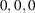that have site-symmetry groups of equal or higher order. Consider, for example, the two general-position diagrams of the space group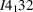(214) shown in Fig. 1.4.2.8. The polyhedra of the left-hand diagram are centred at special points of highest site-symmetry, namely, at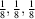and its equivalent points in the unit cell. The site-symmetry groups are of the type 32 leading to polyhedra in the form of twisted trigonal antiprisms (cf. Table 3.2.3.2). The polyhedra (sphenoids) of the right-hand diagram are attached to the originand its equivalent points in the unit cell, site-symmetry group of the type 3. The fractions attached to the polyhedra indicate the heights of the high-symmetry points along the projection direction (cf. Section 2.1.3.6for further explanations of the diagrams).Figure 1.4.2.8 | top | pdf |General-position diagrams for the space group(214). Left: polyhedra (twisted trigonal antiprisms) with centres atand its equivalent points (site-symmetry group .32). Right: polyhedra (sphenoids) attached toand its equivalent points (site-symmetry group .3.).

References

Müller, U. (2012). Personal communication.
Suescun, L. & Nespolo, M. (2012). From patterns to space groups and the eigensymmetry of crystallographic orbits: a reinterpretation of some symmetry diagrams in IUCr Teaching Pamphlet No. 14. J. Appl. Cryst. 45, 834–837.